# McRuffy Press Blog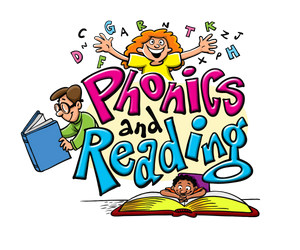## The Role of Systematic Phonics Instruction in Phonemic Development

The Role of Systematic Phonics Instruction in Phonemic Development

by Brian Davis

Phonics instruction is a vital part of a successful literacy program.  Students should receive direct and systematic instruction in the basics of decoding.  This is particularly important in the primary grades.  Increased knowledge of the phonetic structure of the English language is helpful to all students and is especially helpful to struggling students.

Palmaffy shares the National Institute of Child Health and Human Development (NICHD) Chief Reid Lyon’s views on the absolute necessity of phonics instruction for half the children learning to read (as cited in Palmaffy, 1997, Phonics ascendant):

The next quartile of children will learn to read, but they may fall behind without strong early phonics instruction.  For the remaining 25 percent, though reading will be one of the greatest challenges they will face in life.  To enable them to meet that challenge, Lyon says “phonics is non-negotiable.”  Without systematic, explicit instruction in the sound-symbol relationships that comprise the English language, they will not read with the facility required to glean meaning from text.

Developmental psychologist Dr. Joseph Torgesen found that children who could not distinguish the 44 phonemes in the English language were almost always unable to read.  He and his colleagues also found that if these same students had received intensive, explicit phonics early intervention instruction, most would be able to read at or near grade level. (As cited in Stephenson & Reynolds, 1998, Phonemes first)

Torgesen’s research group completed a two-and-a-half-year-long study in 1996 involving 180 kindergarten children who were identified to be at risk of reading failure by the second grade.  The group that received explicit phonics instruction had a 9 percent retention rate compared to 41 percent for the group that did not.

The researchers conducted a follow-up study with fourth and fifth grade students described as being severely dyslexic by their teachers.  The students received a total of 67 hours of intensive phonics training over a two-month period.  All the students made huge gains in their ability to read.  (As cited in Stephenson & Reynolds, 1998, Early intervention the key)

Even widely used programs such as Reading Recovery are much more successful when explicit phonics instruction is included.  “Tunmer found that when Reading Recovery was modified to be more systematic, it was 37% more effective.” (as cited in Grossen, Coulter & Ruggles)  A research study commissioned by the New Zealand Ministry of Education found that “the failure of RR to significantly improve the reading performances of children in the present study is most likely due to the instructional philosophy and practices of RR.” The researchers specifically cited the cause of this failure was the lack of recognition that “skills and strategies involving phonological information are of primary importance in beginning literacy development.”  (Chapman, Tunmer, & Prochnow, 1996)

Yet, Askew, Pinnel, Fountas, Schmitt, and Lyons state that Reading Recovery does give “specific and explicit attention to letters, sounds, and words, both while reading and writing extended text and as direct instruction.” (as cited in Robinson, McKenna, and Wedman, 2000, p. 296)  The important thing to note is that both critics and proponents of Reading Recovery acknowledge that phonics instruction is a vital part of a program to help remediate reading difficulties.

Instructional practices involving the teaching of phonological processing is gaining much more attention.  While not neglecting the need to teach other reading skills, the importance of systematic phonics instruction was highly emphasized in the findings of the National Reading Panel.  “Overall, the findings showed that teaching children to manipulate phonemes in words was highly effective under a variety of teaching conditions with a variety of learners across a range of grade and age levels…” (National Institute of Child Health and Human Development, 2000, Findings and determinations)

Still, many questions remain for educators.  What phonics approach is best?  How much time should be spent on phonics instruction?  How should phonics fit into a balanced literacy program?

One distinction in methodologies is between analytic and synthetic approaches.  A synthetic approach starts by teaching children isolated sounds.  Those sounds are then blended (synthesized) into words.  The National Reading Panel found that that systematic synthetic phonics instruction was of particular benefit to students with learning disabilities, low-achieving students, and low socioeconomic status students. (National Institute of Child Health and Human Development, 2000, Findings and determinations)

In an analytic approach, students begin with known words.  The letter-sound relationships in those words are analyzed.  This is a whole-to-parts method.  Some analytic methods are systematic, while others may take a more intrinsic approach, teaching phonics concepts as the need arises.

Often, isolated sounds are slightly modified when combined with other sounds.  McCormick (1999, p. 302) states that “those who subscribe to this method of presentation maintain that letter sounds should be produced only within the context of words because some sounds are difficult to pronounce in isolation.”   The Latin root of the word “consonant” means “to sound with”.  Indeed, some consonants are difficult to pronounce in isolation without adding a vowel sound; /b/ is not /bu/.  B-a-t is not blended into /bu-at/.

Analytic methods can put more phonemic demands on the students. The advantage of the synthetic approach is that students do not need to have as strong of phonemic segmentation skills.  That is, they do not have to know how to break words down into sounds.  In fact, the synthetic approach teaches this skill.

Dare cites a study of 13 primary classrooms. (Dare, 1999, Sound out words) The classes were divided into three groups.  Four classes were taught by an analytic phonics method.  Four classes were using a combination of analytic phonics and phoneme-and-rime awareness training.  The last five classes were taught using a synthetic phonics approach.  By March, the synthetic group was reading eight months ahead of the other two groups.

There are other methods that fall somewhere between these two basic approaches.  Another common phonics instructional method involves using word families.  Students draw upon their previous knowledge to expand their word knowledge base.  Common words are divided between the onset and the rime. The onset is the part of the word that comes before the vowel.  The rime is the rest of the syllable.

Activities are designed to manipulate words by substituting different onsets to make new words with the rime.  For example, the word cat can be changed to bat, hat, and sat by changing the onset.  Students may practice this using magnetic letters, tiles, or erasable boards.

In my personal experience, I have used all of these methods to some extent.  In my kindergarten classes, students were rapidly introduced to the short a sound and seven consonants in a five-week period.  The consonants were chosen by how well they could be used to form common words with the short a vowel sound.

Students were taught the sounds in isolation, but within the same lesson, the new letters were formed into words using previously learned sounds.  With the introduction of a limited sight vocabulary (the, is, in, and on) students began to write and read these words in the context of sentences and stories featuring decodable text.  As new sounds were introduced, rime-onset activities explored new words.

Classroom averages of students taught with this methodology were in the 99th percentile on the language arts sections of the Iowa Tests of Basic Skills.  By the end of first grade, one of my former students scored at the 6.9 grade level on the SAT.  One parent was particularly surprised when his kindergarten son opened a menu in a restaurant and began to read it.

A key component to success is connecting phonetic instruction to actual reading experiences.  Honig states: “Connected reading provides the meaningful exercises necessary for linking spelling patterns with the rest of the cognitive system, whereas phonics without connected reading amounts to useless mechanics and is too easily forgotten.” (Honig, 1996, p. 66-67)

Using phonetic knowledge in connected text builds fluency. Books can be reread to provide even more exposure to phonics concepts. Students should be given opportunities and encouragement to use their phonics skills in creative writing. Reading, writing, and skill development are all important parts of a balanced literacy approach.

The McRuffy Press Kindergarten SE program uses reading material that is highly connected to the phonics concepts.  Themes within the books are used to develop writing activities.  Students are even encouraged to interact with the stories using creative drama.  Students also practice spelling, grammar, handwriting, and other basic skills integrating phonics concepts.

Teachers could further develop a balanced literacy approach from this foundation by reading and discussing a wider variety of literature.  These in turn could be a part of guided reading and writing experiences.  Teachers could further reinforce phonics concepts by pointing out words that fit the current phonics concepts in the shared-reading of big books.

Some researchers feel that “…too much attention to phonics can detract from the construction of meaning…”  (Weaver, 1996 Toward a consensus on teaching phonics) Integrating the phonics concepts into spelling, reading, language, and writing greatly increases the exposure to the phonics concepts.  This eliminates the need for extensive drilling periods.  One of the other benefits is that it puts the phonics concepts into a meaningful context that enables students to develop comprehension skills along with phonics skills.

In my teaching experience, I have noticed that as students gain competency in phonics, less effort is spent on decoding.  This allows children to focus more on context and meaning.  The labored blending of consonants and vowels is replaced by instantaneous word recognition and fluent reading.  This is often referred to as automatic recognition.  I believe this to be due in part to following a prescribed sequence of instruction.

Allington (Allington, n.d.) concludes that: “There is no “scientifically” determined sequence of instruction and no conclusive evidence on what sorts of phonics lessons, of what duration would most effectively develop the optimum level of decoding efficiency in children.”  Perhaps this is due to the self-evident structure of the English language.  A logical progression would be from simple common words to more complex word structures.

Progression from exposure to regular spellings of phonemes to irregular spellings would be the next step.  Phonics instruction in the later primary grades and above should focus more on larger parts of words (affixes, morphemes, root words, syllabication).  Chall & Popp outline a general format for a primary level progression of systematic phonics instruction (Chall & Popp, 1996, chap. 7).  They advocate teaching consonants before vowels, short vowel words before long vowel words ending with silent e, then other long vowel words, and finally syllabication.

Exposure to good systematic phonics instruction should help students develop the cognitive structures that will allow them to make generalizations.  Once students understand the underlying concepts that words are composed of bits of sounds (phonemes) that can be decoded and encoded using symbols (graphemes),  phonics instruction can be as simple as telling the child that “ai makes the long a sound in the words rain, train, and brain.”

Most of my students reached the point where they could make these generalizations independently by the end of Kindergarten (some by mid-year).  Yet, systematic phonics instruction was still helpful in developing an understanding of spelling patterns.  It also highlights variations in grapho-phonemic patterns.

Through a systematic presentation of phonics concepts, I was able to ensure that all students gained a foundational skill for reading that allowed them to progress in developing other reading and writing skills.  Although students progressed at various rates, they all seemed to benefit from the initial instruction.  Some students required additional reinforcement and practice to address specific weaknesses.  But, seeing the differences between what was initially taught and what was learned helped to refine the instructional focus to meet individual needs.

Phonics knowledge and skills alone are not enough to achieve literacy, yet it is vital to reading success.  Instruction in phonics does not need to “drill and kill” interest in reading.  It can be a vital part of a balanced literacy program even if taught systematically and directly.   Activities can be enjoyable and relate directly to contextual reading.

Research, debates, and commentaries on the topic of phonics instruction are filled with accusations of misinterpretation of “scientific evidence”.  It is at times emotionally charged.  Phonics and reading instruction has ridden political and philosophical pendulums.  The issues related to the role of phonics in reading instruction are by no means resolved either in the scientific literature or in practice.  As long as educators strive for the best interests of the learner, this on-going dialog should encourage us to keep seeking and keep learning, and that will ultimately produce a very positive outcome.

Allington, R.L. (n.d.) Overselling phonics.  Retrieved March 25, 2002, from University at Albany State University of New York Web site: http://www.albany.edu/reading/

allington/phonics.htm

Chall, J. S., Popp, H. M. (1996) Teaching and assessing phonics: Why, what, when, how. Cambridge, MA: Educators Publishing Service, Inc.

Chapman, J., Tunmer, W., Prochnow, J. (1996) Success in Reading Recovery depends on the development of phonological processing skills.  [Electronic version] Massey University, New Zealand Retrieved March 19, 2002, from http://www.nrrf.org/

rr_study_chapman.htm

Dare, M. (1999) Phonics works: But some programs work better than others. [Electronic version].  Professionally Speaking, June 1999.  Retrieved March 19, 2002 from http://www.oct.on.ca/english/ps/june_1999/phonics.htm

Davis, B. (2014) McRuffy Kindergarten SE Phonics and Reading. Raymore, MO: McRuffy Press https://www.mcruffy.com

Grossen, B., Coulter, G., Ruggles, B. (1996) Reading Recovery: An evaluation of benefits and costs.  Effective School Practices, 15 (3).  Retrieved July 15, 2000

Honig, B. (1996) Teaching our children to read. Thousand Oaks, CA: Corwin Press, Inc.

McCormick, S. (1999) Instructing students who have literacy problems. Upper Saddle River, NJ: Prentice-Hall.

National Institute of Child Health and Human Development. (2000)  Teaching children to read:  An evidence-based assessment of the scientific research literature on reading and its implications for reading instruction.  (NIH Pub. No. 00-4769) Retrieved July 21, 2000 from http://www.nichd.nih.gov/publications/nrp/findings.htm

Palmaffy, T. (1997). See Dick Flunk [Electronic version].  Policy Review, 86. Retrieved February 10, 2002, from http://www.policyreview.org/nov97/flunk.html

Askew, B. J., Pinnell, G. S., Fountas, I. C., Schmitt, M. C., & Lyons, C. A. (1998) Reading recovery review: Understandings, outcomes, and implications. In R.D. Robinson, M. C. McKenna, J. M. Wedman (Ed) Issues and trends in literacy education (Second edition). Needham Heights, MA: Allyn & Bacon

Stephenson, F & Reynolds, A.  (1998/1999 Fall & Winter) The Phonics Revival {Electronic Version].  Research in Review.  Retrieved February 10, 2002, from http://www.research.fsu.edu/researchr/fallwinter9899/features/phonics.html

Weaver, C. (1996) Facts on research on the teaching of phonics. [Electronic version] Prepared for the Michigan English Language Arts Framework project.  Retrieved March 25, 2002, from http://www.ncte.org/wlu/08894f2.htm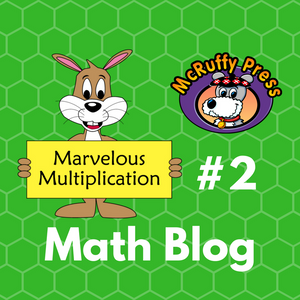## Marvelous Multiplication #2

Marvelous Multiplication Trick #2: A Multiplication Challenge Using Easy Squares.

If you haven’t already, check out our previous three blogs:

Quick Math: Find the Squares of Numbers That End With 5

Quick Math: Squaring Numbers 51 to 59

In the previous post (Marvelous Multiplication #1) we generated and easily solved problems based on what we termed “easy squares” of two-digit numbers. This included the techniques learned in the two Quick Math blogs and squares of multiples of ten (10, 20, 30, 40…). We generated multipliers by adding and subtracting 1 to and from the easy squares.

In this post, we’ll expand the number of multiplication problems that can be generated and suggest a math challenge game.

The Trick:

Generate 2 two-digit multipliers around an easy square by adding and subtracting the same number to and from the easy square. In the last post, we subtracted 1, but the trick works with adding and subtracting any number. Let’s use the easy square of 30 for an example and add and subtract 2 for the multipliers. We’ll call the number 2 the “distance” between either multiplier and the easy square.

30 -2 = 28 and 30 + 2 = 32 Our multipliers will be 28 and 32.

To quickly find the product of 28 x 32 find the product of the easy square (302 = 900) and subtract the square of the distance. (22 = 4)

28 x 32 = 900 – 4 = 896

Let’s try it with a “distance” of 3:

30 – 3 = 27 and 30 + 3 = 33 Our multipliers will be 27 and 33.

27 x 33 = 900 – 9 = 891

Teaching Sheet 1 provides a form children can use to practice stepping through the process. Chose an easy square and write the digits in the boxes. Choose a “distance” number. Add and subtract the “distance” to generate multipliers for the problem.

Write the product of the easy square on the bottom row and the distance square. Subtract the distance squared to find the answer.

Challenge Game:

Given a two-digit number, generate a problem around an Easy Square and use the math tricks to solve the problem. The ultimate goal would be doing all the steps using mental math, but teaching sheets 2 and 3 present the steps involved in solving the problems to practice thinking through the steps.

For example, try the number 43.

You can make an easy square based problem around the Easy Square of 45. The distance between 43 and 45 is 2. (45 – 43 = 2) The second multiplier will be 47 (45 + 2 = 47). The problem generated will be 43 x 47.

The Easy Square of 45 is 2025. The Distance of 2 squared is 4.

2025 – 4 = 2021 so 43 x 47 = 2021

You could also make an easy square based problem around the Easy Square of 40. The distance between 43 and 40 is 3. (43 – 40 = 3) The second multiplier will be 37 (40 - 3 = 37). The problem generated will be 43 x 37.

Easy Square is 402 = 1600 and the Distance squared is 32 = 9

43 x 37 = 1600 – 9 = 1591

You could also make an easy square based problem around the Easy Square of 53. The distance between 43 and 53 is 10. The second multiplier will be 63. The problem generated will be 43 x 63.

The Easy Square is 532 = 2809 and the Distance squared is 102 = 100

43 x 63 = 2809 – 100 = 2709

Sheet 2 shows all the steps. Start with a random two-digit number and write it on the top line of the “Generate a Problem” section. Choose a nearby easy square. Write the number that is to be added or subtracted to equal the easy square. Next, write the easy square. Generate the second multiplier by doing the opposite operation to the Easy Square. Write the second multiplier in the boxes.

On the bottom row write the products of the squares of the Easy Square and Distance. Subtract for the answer to the generated multiplication problem.

Sheet 3 visually simplifies the process. The first section is used to write the multiplication problem starting with any given two-digit number. The ES boxes are for the Easy Square. The D boxes are for the Distance. Most of the time it will be a single digit distance, but it can be any number of digits. The sheet is set up for a distance with a maximum of two-digits. The far right boxes are for the squares of the Easy Square and Distance.

Encourage children to skip any boxes they can do mentally. For example, if they can write the product of the Easy Square directly in the far right boxes, skip the center box. If the distance and distance square are smaller numbers and easy to remember those boxes can be skipped.

Why it Works:

Let’s build an algebra problem. We’ll represent the Easy Square with the letter a and the “distance” with the letter b. We generated the multipliers by adding and subtracting the “distance” to and from an Easy Square.

(a – b)(a + b) = a2 + ab – ab – b2 =  a2 – b2

This is true for any “distance” even when it makes numbers negative and multipliers have more than two digits. For example, with the number 30 we can make the “distance” 200.

The multipliers are 230 and -170

Easy Square is 302 = 900 and The distance squared is 2002 = 40,000

230 x (-170) = 900 - 40,000 = -39,100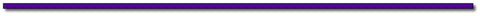## Thanks for Visting Savings:

Use the code MarMult2 to save \$3.00 off every Color Math Curriculum Package. See eligible products here.

Offer Expires July 30, 2017Subscribe to this blog's RSS feed using http://feeds.feedburner.com/McruffyPress-McruffyMathBlog## McRuffy Press offers complete math curriculum by grade level, math activity books, manipulatives, games and more! Find more McRuffy Math products here.

Improve multiplication facts skills with our Multiplication Fast Fact books: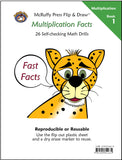Multiplication Facts (Book 1) Drills factors from 1 to 10 in our self-checking, non-consumable format that allows students to practice until they have mastered basic facts.Multiplication Practice (Book 2) Drills factors from 1 to 10 in our self-checking, non-consumable format that allows students to practice until they have mastered basic facts.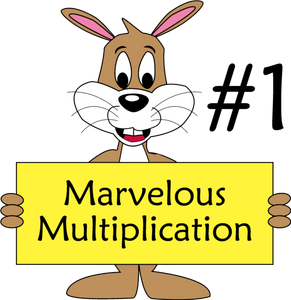## Marvelous Multiplication #1

Marvelous Multiplication Trick #1: Using Math Squaring Tricks to Multiply Other Numbers.

If you haven’t already, check out our previous two blogs:

Quick Math: Find the Squares of Numbers That End With 5

Quick Math: Squaring Numbers 51 to 59

We can use these techniques to do some Quick Math multiplying two-digit numbers that aren't squares. The tricks in this post offer more of an entertainment value than everyday practical value. Helping children feel empowered to do math is a great thing. These tricks do that! Once after teaching these techniques to some children, they replied, “Wow! That’s what algebra does.”

Like the previous two tricks, the minimum a child has to understand is basic multiplication facts. The Why it Works section is great to help students who have some understanding of algebra see how math mysteries can be resolved.

The Trick:

We’ll start with the example of 64 x 66. After learning the trick you’ll immediately see that the product is 4224. First, let’s explore lists of problems we can use for the trick.

We can apply the trick to all these problems:

14 x 16, 24 x 26, 34 x 36, 44 x 46, 54 x 56, 64 x 66, 74 x 76, 84 x 86, 94 x 96

Do you see the pattern?

It also applies to these problems:

50 x 52, 51 x 53, 52 x 54, 53 x 55, 54 x 56, 55 x 57, 56 x 58, 57 x 59, 58 x 60, 59 x 61

And these problems:

19 x 21, 29 x 31, 39 x 41, 49 x 51, 59 x 61, 69 x 71, 79 x 81, 89 x 91

The lists of problems do not need to be memorized because there is a simple method to generate the problems:

Start with a number that the child has learned to easily square. We’ll call a number to be squared an Easy Square.

The problems were generated by adding and subtracting one from the numbers that were squared. For example, start with the Easy Square of 65.

Generate the problem by subtracting and adding one: (65 – 1)(65 + 1) = 64 x 66.

The solution is simply the product of the Easy Square minus 1. Applying the Quick Math trick from the first blog we can quickly find the solution. 4225 – 1 = 4224.

The middle row of problems was generated from the Easy Squares demonstrated in the second blog.

For example, using the trick we can quickly know that 532 = 2809. From that we generate the problem 52 x 54 = 2809 – 1 = 2808

We can call the squares of two-digit numbers ending with zero Easy Squares, too. That was how we generated the last row of problems with 9’s and 1’s in the units place.

For example: 802 = 6400, so 79 x 81 = 6400 – 1 = 6399.

Practice the trick, and we’ll explore how to greatly expand the list of problems that can be generated in our next blog.

The Teaching Sheet provides a form children can use to practice stepping through the process. Chose an easy square and write the digits in the boxes. Skip to the other side of the page and generate a -1, +1 problem. Use an easy square trick to find the square of the Easy Square. Write the answer in the Th, H, T, O boxes. Subtract 1 to find the product of the generated problem.

Why it Works:

Let’s build an algebra problem. We’ll represent the Easy Square with the letter a. We generated the multipliers by adding and subtracting one to an Easy Square.

(a – 1)(a + 1) = a2 + a – a – 12 =  a2 – 1

The algebra problem shows we can substitute any of our Easy Square numbers. Apply the easy square trick and subtract one.

The formula is not limited to two-digit numbers. For example:

2002 = 40,000    So 199 x 201 = 39,999

Nor is it limited to Easy Squares. It’s just more difficult to apply. For example generating a problem from 392:

38 x 40 = 392 -1  = 1521 – 1 = 1520. It’s actually easier to multiply 38 x 40 than to first square 39 and then subtract one, so the trick just isn’t helpful. Nevertheless, there is a trick involving this problem, but that’s for another blog.## Thanks for Visting Free Gift:

Offer Expires July 15, 2017Subscribe to this blog's RSS feed using http://feeds.feedburner.com/McruffyPress-McruffyMathBlog## McRuffy Press offers complete math curriculum by grade level, math activity books, manipulatives, games and more! Find more McRuffy Math products here.

Improve multiplication facts skills with our Multiplication Fast Fact books:Multiplication Facts (Book 1) Drills factors from 1 to 10 in our self-checking, non-consumable format that allows students to practice until they have mastered basic facts.Multiplication Practice (Book 2) Drills factors from 1 to 10 in our self-checking, non-consumable format that allows students to practice until they have mastered basic facts.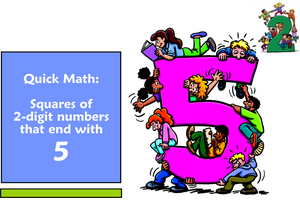## Quick Math: Find the Squares of Numbers That End With 5

Want to teach your child some amazing math shortcuts? Follow our new blog series. The first blog features a math trick to quickly square numbers that end with 5.

It’s simple enough for children who have knowledge of basic multiplication facts.

For younger children simply teach the trick. For students learning algebra, explore in the Why it Works section for the more mathematically curious. In future blogs, we’ll do some interesting things with this trick to multiply numbers that aren’t squares.

The Trick:

You can quickly find the square of any two digit number ending with 5, such as 15, 25, 35, 45, etc. The trick actually works for any number that ends with five, but the mental math becomes increasingly difficult with numbers greater than 95.

Simply look at the digit in the tens place and multiply by the next digit. The product will be the digits for the thousands and hundreds place (Note the thousands place will be zero for 152 and 252). Add 25 to that product for the product of the number squared.

For example: 352

Multiply the number in the tens place, 3 by the next number, 4.

3 x 4 = 12 the product is the thousands and hundreds place digits. The 12 represents 1200. Then add 25:

352 = 1225

Print Teaching Sheets for the trick. On the first two sheets ask students to put in a random digit in the first box to choose a number to square. Students use that digit and that digit + 1 to make a multiplication problem for the thousands and hundreds place values. If the product is a single digit, the thousands place is left blank (152 and 252). The third page is a practice sheet for the numbers 15 to 95.

Why it works:

Think of any square of a two digit number as the square of a sum of the tens and ones places.

Let’s make the tens place X and the units (ones) place Y.

So, for the number 35,  x = 30 and y = 5

The square of 35 is the square of our x and y values. Let’s work with the variables first.

(x + y)2 = (x + y)(x + y) = x2 + xy + xy + y2 = x2 + 2xy + y2

When there are 5 ones (y = 5) then 2xy is 10x .

So the problem simplifies to x2 + 10x + y2   Furthermore, y2 will be 52 = 25

So, x2 + 10x + 25

We can group using the associative property and factor:  (x2 +10x) + 25  = x(x +10) + 25

X+10 is the current tens place plus ten more, which is the next ten. In our example, it will be 30 and ten more, 40. So the product of X times the next ten in our example is 1200.

Add 25 to 1200 in our example of 352 = 1200 + 25 = 1225.

Try it with all the squares that end with 5 from 15 to 95.

152 = 225
252 = 625
352 = 1225
452 = 2025
552 = 3025
652 = 4225
752 = 5625
852 = 7225
952 = 9025

You may continue forever, but the mental math becomes more cumbersome. For 1052 The product of 10 x 11 generates the ten thousands, thousands, and hundreds places. Tens and Units will still be 25

1052 = 11025
1152 = 13225## Thanks for Visting Free Gift:

Offer Expires June 30, 2017Subscribe to this blog's RSS feed using http://feeds.feedburner.com/McruffyPress-McruffyMathBlog## McRuffy Press offers complete math curriculum by grade level, math activity books, manipulatives, games and more! Find more McRuffy Math products here.

Improve multiplication facts skills with our Multiplication Fast Fact books:Multiplication Facts (Book 1) Drills factors from 1 to 10 in our self-checking, non-consumable format that allows students to practice until they have mastered basic facts.Multiplication Practice (Book 2) Drills factors from 1 to 10 in our self-checking, non-consumable format that allows students to practice until they have mastered basic facts.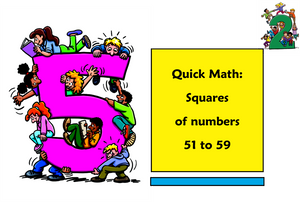## Quick Math: Squaring Numbers 51 to 59

In our previous post, we found a quick shortcut to square two-digit numbers with 5 in the ones place. There is an even simpler shortcut to square two-digit numbers with the digit 5 in the tens place.

For younger students, you can simply teach the trick. For older students explore the Why It Works  section.

The Trick:

To get the thousands and hundreds place value add the digit in the ones place (units) to 25.

To get the tens and ones place, square the number in the ones place. The tens place will be zero for the numbers 51, 52, and 53.

For example, 542 Add the ones digit to 25 to get the thousands and hundreds places. 25 + 4 = 29, which represents 2900.

Square the digit in the ones place to get the digits for the tens and units. 4 x 4 = 16

542 = 2900 + 16 = 2916

Print the Teaching Sheets for the trick. On the first sheet ask students to put in a random digit in the box to complete the two-digit number to square. Follow the steps. Remind students that if the product of squaring the ones digit is a single digit answer, the tens place is zero.

The second page is a practice sheet for the numbers 51 to 59.

Why It Works

Like the previous post, let’s make the tens place X and the units (ones) place Y and solve for variables first. The algebra is the same equation as last time. In fact, this algebraic equation is the same for the square of any two-digit number.

(x + y)2 = (x + y)(x + y) = x2 + xy + xy + y2 = x2 + 2xy + y2

In our example of 542,  x = 50 and y = 4

The 25 that we start with for the thousands and hundreds place is simply x2 .

Since all the numbers from 50 to 59 have the digit 5 in the tens place, it will be 25 for all the numbers. Remember this is actually 50 times 50 which equals 2500.

The step for adding the units digit to 25 comes from the 2xy. We can use the associative property of multiplication to first find the product of 2x and then multiply by y. For all the numbers in the 50's, x=50 Two times that will equal 100. 2(50) = 100.

So the 2xy part of the algebra equation will always yield a product that puts the units digit into the hundreds place.

For our example of 54 x = 50, y = 4, so 2xy = (two times 50) times y. Which is 100 times y.

100 times 4 is 400.

Add that to the  x2 which will be 2500. 2500 + 400 = 2900

Next, add the digits for the tens and units. This is the ypart of the algebra equation. Remember that we designated y as the units place. In our example of 54, the units (ones) place is 4, so we square that number and add it to 2900 for the square are 54.

y= 4 x 4 = 16

54= 2900 + 16 = 2916.

Try it with all the squares that begin with 5 from 50 to 59.

502 = 2500
512 = 2601
522 = 2704
532 = 2809
542 = 2916
552 = 3025
562 = 3136
572 = 3249
582 = 3364
592 = 3481## Thanks for Visting Free Gift:

Practice Multiplication Skills with Multiplication Sliders  Download Here and use the discount code: MathBlog at checkout and receive your PDF file instantly! Offer Expires June 30, 2017

Offer Expires June 30, 2017Subscribe to this blog's RSS feed using http://feeds.feedburner.com/McruffyPress-McruffyMathBlog## McRuffy Press offers complete math curriculum by grade level, math activity books, manipulatives, games and more! Find more McRuffy Math products here.

Improve multiplication facts skills with our Multiplication Fast Fact books:Multiplication Facts (Book 1) Drills factors from 1 to 10 in our self-checking, non-consumable format that allows students to practice until they have mastered basic facts.Multiplication Practice (Book 2) Drills factors from 1 to 10 in our self-checking, non-consumable format that allows students to practice until they have mastered basic facts.